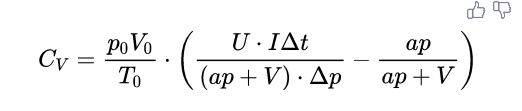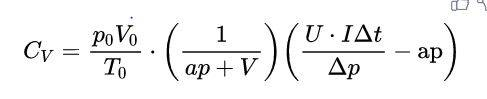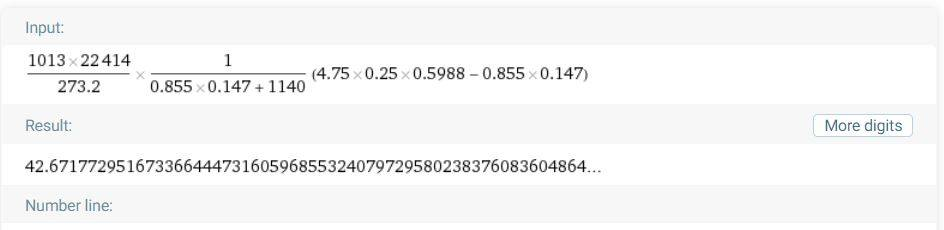# Need help with calculating a Cv in PHYWE's Heat Capacity of gases

TechieDork
Homework Statement:
-Calculating a Cv from the given equation and data , if everything goes right you'll obtain Cv=26 J*K^-1*mol^-1 as a result.
Relevant Equations:
$$C_{V}=\frac{p_{0} V_{0}}{T_{0}} \cdot\left(\frac{U \cdot I \Delta t}{(a p+V) \cdot \Delta p}-\frac{a p}{a p+V}\right)$$
I've conducted this experiment yesterday. The main goal of this experiment is to find a gas constant R and compare it with its theoretical counterpart but I get stuck in calculating a Cv so I tried to find out what's wrong with my calculations by trying to calculate a Cv from the given data....(1)

where p0 = 1013 hPa , T0=273.2 K , V0 = 22414 ml/mol
U = 4.75 V , I = 0.25 A
a = 0.855 cm^3/hPa
p = 0.147 hPa
V = 1.14 L = 1140 mL
delta(p)/delta(t) = 1.67 hPa/s or delta(t)/delta(t) = 0.5988 s/hPa

If everything goes right I'll obtain Cv = 26 J*K-1*mol^-1 as a result

My attempt :...(2)...(3)

Plugged all the given values into the eq(2) and I got 42.67 instead of 26.

What is wrong with my calculation? , could someone please shed some light on this?

Here this is the documentation : https://www.nikhef.nl/~h73/kn1c/praktikum/phywe/LEP/Experim/3_2_02.pdf (page 4 and 5)

#### Attachments

Last edited:

Homework Helper
Are your units consistent? Try converting everything to SI units.

•TechieDork
TechieDork
Are your units consistent? Try converting everything to SI units.

I have tried a dimensional analysis with my units and yes...they are indeed consistent in the form of (J*K^-1*mol^-1).

I tried converting all of them to their SI units and plugged them in the equation but the result doesn't get anywhere near 26.
I think I'm getting stuck in the endless loop now. Could anyone please show me how?

Last edited:
Gold Member
I think you are mistaking ##p## and ##\Delta p## and and are substituting the wrong value for ##p##. According to the documentation, ##p=1011~\rm{hPa}## (see page 5).